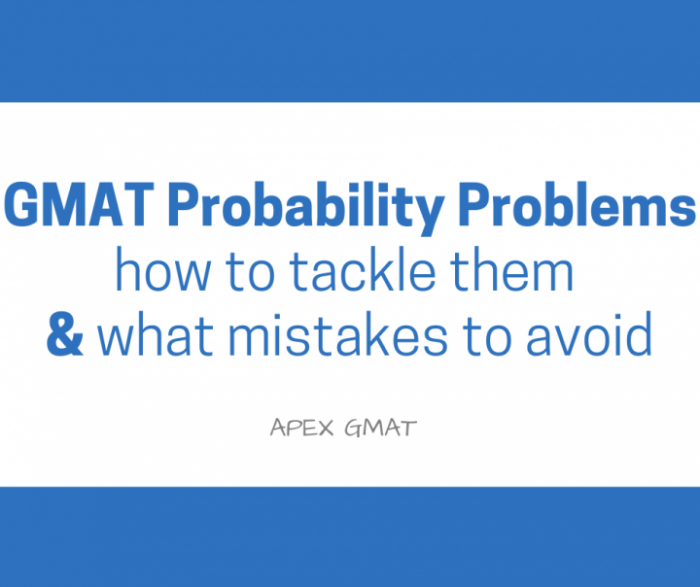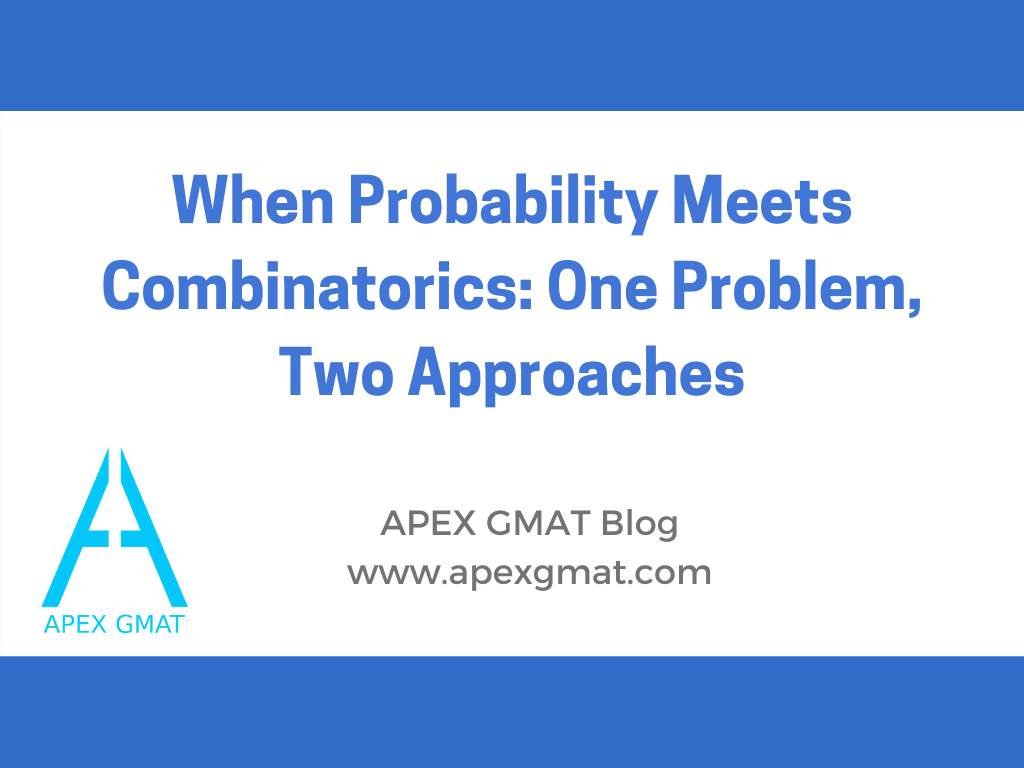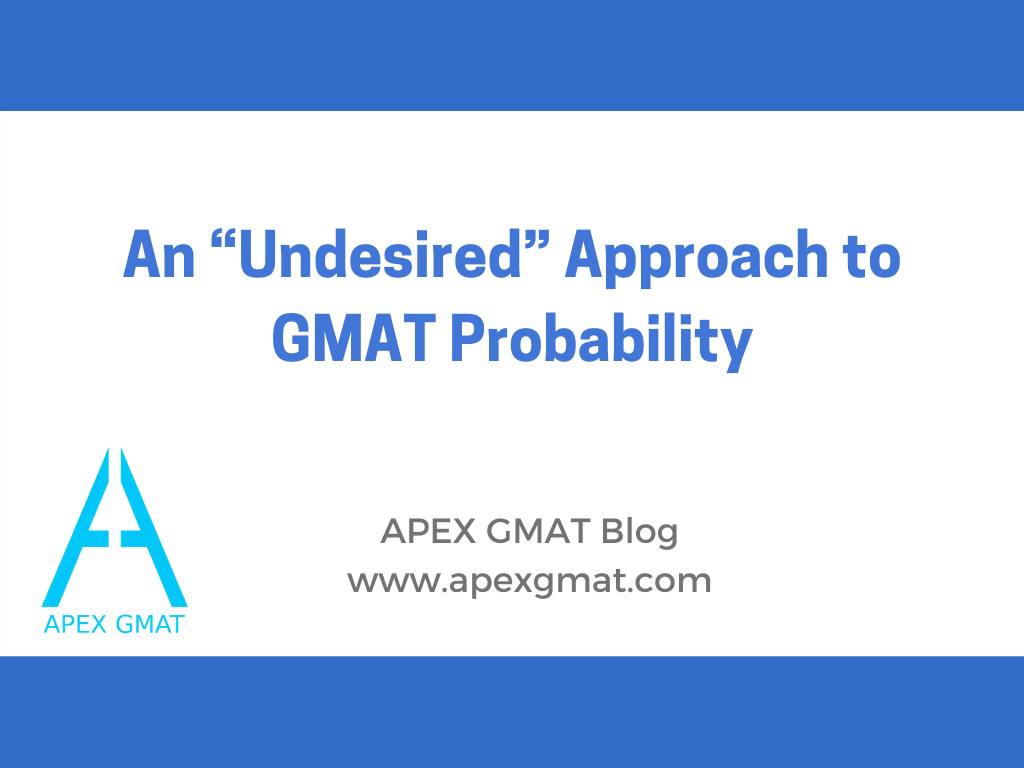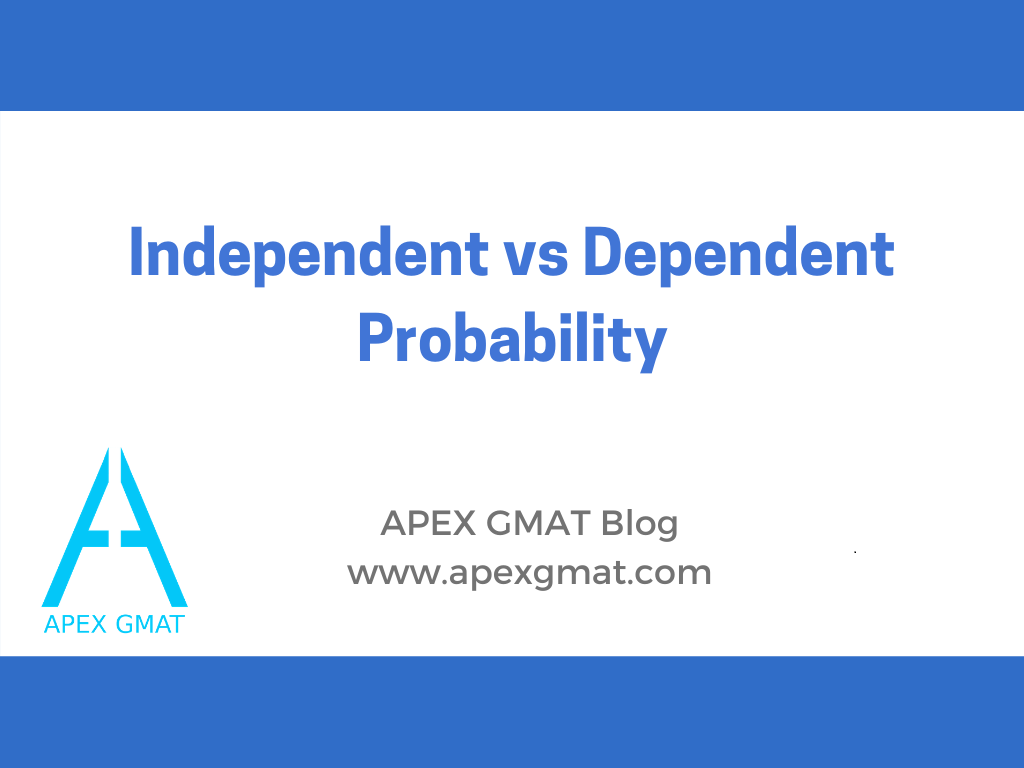## GMAT Probability Problems – How to Tackle Them & What Mistakes to Avoid

By: Apex GMAT Contributor: Ilia Dobrev Date: August 12, 2021 The concept of probability questions is often pretty straightforward to understand, but when it comes to its application in the GMAT test it may trip even the strongest mathematicians. Naturally, the place...## When Probability Meets Combinatorics: One Problem, Two Approaches

By: Rich Zwelling, Apex GMAT Instructor Date: 16th March, 2021 Now, we’d like to take a look at an Official GMAT Probability problem to pull everything together. The following is a good example for two reasons:  1. It illustrates a quirky case that is difficult more...## An “Undesired” Approach to GMAT Probability

By: Rich Zwelling, Apex GMAT Instructor Date: 11th March, 2021 In our last post, we discussed a solution for the following question, which is a twist on an Official Guide GMAT probability problem: Xavier, Yvonne, and Zelda individual probabilities for success on a...## Independent vs. Dependent Probability

By: Rich Zwelling, Apex GMAT Instructor Date: 8th March, 2021 Independent vs. Dependent Probability As promised last time, we’ll return to some strict GMAT probability today. Specifically, we’ll discuss the difference between independent and dependent probability....## What Happens When Permutations Have Repeat Elements?

As promised in the last post, today we’ll discuss what happens when we have a PERMUTATIONS situation with repeat elements. What does this mean exactly? Well, let’s return to the basic example in our intro post on GMAT combinatorics: If we have five distinct paintings,...